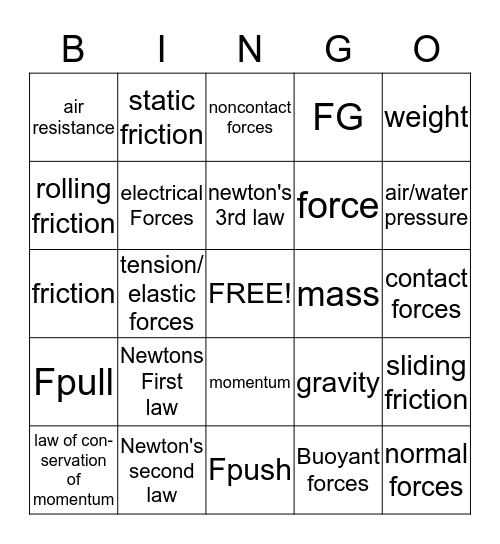# ForcesThis bingo card has a free space and 24 words: Newtons First law, Newton's second law, newton's 3rd law, law of con- servation of momentum, weight, air resistance, rolling friction, static friction, mass, momentum, force, friction, gravity, sliding friction, electrical Forces, Buoyant forces, contact forces, noncontact forces, air/water pressure, tension/ elastic forces, normal forces, Fpull, FG and Fpush.

## Play Online